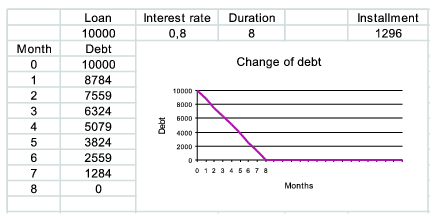Mathematical and Physical Journal
for High Schools
Issued by the MATFUND Foundation
 Already signed up? New to KöMaL?

# KöMaL Problems in Informatics, December 2005

Show/hide problems of signs:## Problems with sign 'I'

Deadline expired on January 16, 2006.

I. 118. We have n ceramic piggy banks and n keys, each corresponding to exactly one of the banks. We throw one key into each bank at random. Then we break open t ceramic pigs. Find the probability that we are able to open the remaining banks using the keys found in the broken ones without any further breaking. Write a program that estimates this probability by making k experiments. If n<10, then we should also compute the exact probability by examining all possible cases.

Your program should read the values of n, t and k from the keyboard, then make the experiments and display the resulting relative frequencies (that is, the ratio of successful outcomes to k), further, the exact probability.

The source code of the program should be submitted (i118.pas, i118.cpp, ...).

(10 pont)

statistics

I. 119. Using the programs Comenius Logo, PC Logo or NetLogo, make an animation showing the classic chess game of Botvinnik and Capablanca at AVRO Championship Rotterdam, 1938.

The size of the board should be 560×560, and each figure should be displayed as the trace of a corresponding turtle.

The final logo project (i119.lgp) should be submitted, containing a procedure chess running the animation.

(10 pont)

solution (in Hungarian), statistics

I. 120. We take a loan of S Euro at p per cent compound interest for n months. We pay back the loan in equal monthly installments: the first installment is paid back at the end of the first month, and theinstallment is at the end of themonth. Before paying back an installment, the bank capitalizes the loan, that is, first our debt is increased according to the rate of interest, only then is the currently paid back sum subtracted from the debt, which is finally rounded up to a nonnegative integer.

Prepare an OpenOffice or Excel sheet summarizing our debt in the course of time. The user enters the initial sum S of the loan, the compound interest rate p and the loan duration n into cells A2, B2 and C2, respectively. Cell E2 should contain the monthly installment, while column B should contain the current debt after paying an installment. Change of the debt should also be graphed in cells C4...F15. In its first row and column some remark should be visible explaining the meaning of the graph.

Example:You should submit the sheet (i120.sxc, i120.xls, ...). The value of n can be assumed to lie between 3 and 24. Cases when the interest rate p is 0 should also be handled correctly.

(10 pont)

solution (in Hungarian), statistics## Problems with sign 'S'

Deadline expired on January 16, 2006.

S. 13. Two players alternately move a piece on the vertices of a directed graph. One loses if he or she cannot move because the current vertex has no outward edge. If the piece is placed for the third time onto the same vertex, the game is a draw. Write a program that determines for each vertex the best possible result of the first player starting from that position.

The program will be run from the command line with two parameters containing the names of the input and output files. The input file contains the graph. The output file should contain whether any given vertex is a winning or a losing one.

The first line of the input file will contain the number of vertices and edges (n,m). The vertices are numbered from 1 to n. The next m lines will contain the origin and endpoint of each directed edge. The output file should have n lines, with theline containing W, D or L, depending on whether the first player starting from thevertex has a winning strategy, both players have a strategy to force a draw, or the second player has a winning strategy, respectively.

The graph can be assumed to have at most 1 000 000 vertices and at most 50 000 000 edges.

Example: the program is run with s13 graph.txt result.txt parameters.

 graf.txt result.txt 5 71 11 22 32 43 14 34 5 DDDWL

The source code of the program (s13.pas, s13.cpp, ...) should be submitted.

(10 pont)

statistics

\$Var(Body)